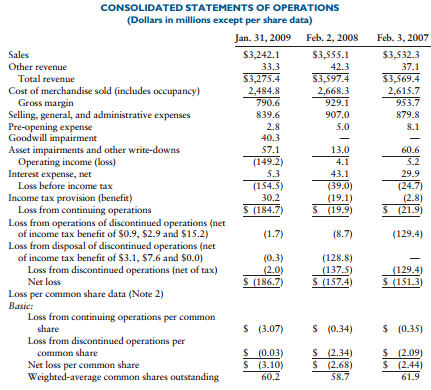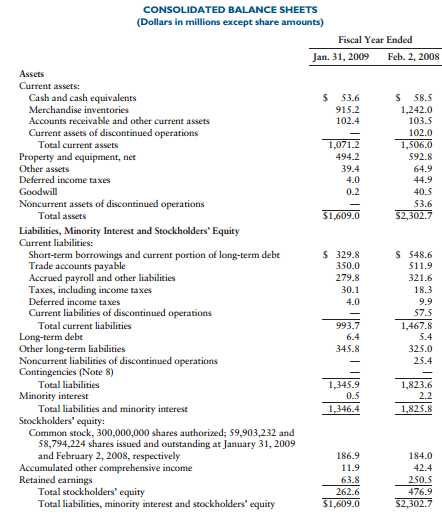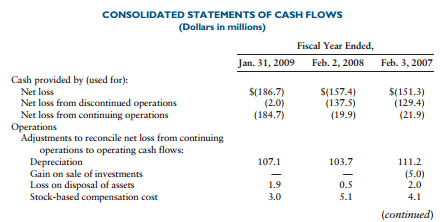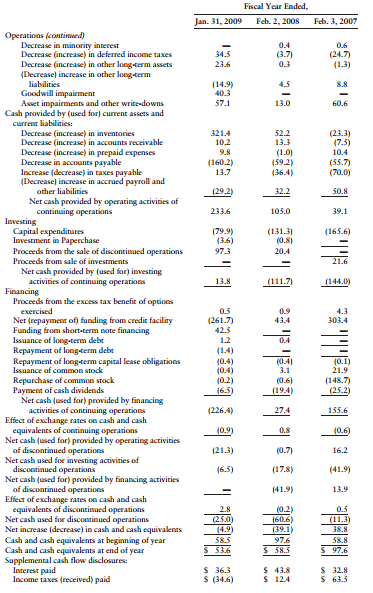### Create an Account

Home / Questions / BOOKS UNLIMITED PART 1 Borders Group Inc presented this information in its 10Ks ...

# BOOKS UNLIMITED PART 1 Borders Group Inc presented this information in its 10Ks Required a Compute the following liquidity ratios for 2009 and 2008 1Days sales in inventory

BOOKS UNLIMITED*—PART 1

Borders Group, Inc., presented this information in its 10-Ks:Required

a. Compute the following liquidity ratios for 2009 and 2008:

1. Days’ sales in inventory

2. Inventory turnover (use ending inventory)

3. Working capital

4. Current ratio

5. Cash ratio

6. Sales to working capital (use ending working capital)

7. Operating cash flow/current maturities of long-term debt and current notes payable

b. Compute the following long-term debt-paying ability for 2009 and 2008:

1. Debt ratio

2. Operating cash flow/total debt

c. Compute the following profitability ratios for 2009 and 2008:

1. Net profit margin

2. Return on assets (use end of year total assets)

3. Return on total equity (use end of year total equity)

4. Gross profit margin

d. Compute or obtain the following investor analysis:

1. Earnings per common share

2. Operating cash flow/cash dividends

e. Comment on the results in (a), (b), (c) and (d).

f. Comment on the trend in net income (loss).

g. Comment on significant trends (items) in the Consolidated Statement of Cash Flows.

h. Using these ratios for 2009 and 2008, comment using the Beaver Study on possible

financial failure:

1. Cash flow/total debt

2. Net income/total assets (return on assets)

3. Total debt/total assets (debt ratio)

Apr 02 2020 View more View LessSubscribe To Get Solution